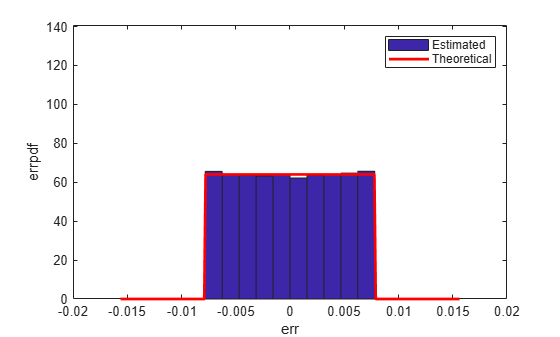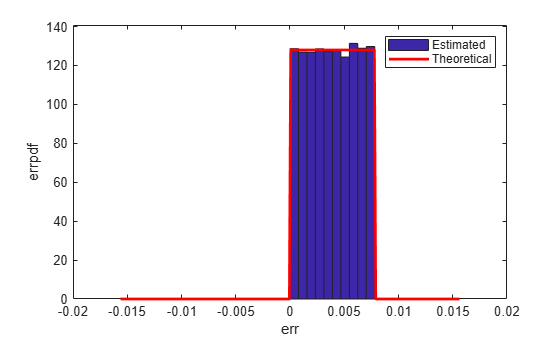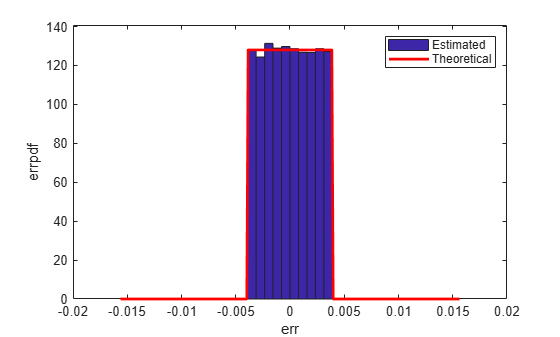# Compute Quantization Error

This example shows how to compute and compare the statistics of the signal quantization error when using various rounding methods. Quantization occurs when a data type cannot represent a value exactly. In these cases, the value must be rounded to the nearest value that can be represented by the data type.

First, a random signal is created that spans the range of the `quantizer` object. Next, the signal is quantized, respectively, with rounding methods `'fix'`, `'floor'`, `'ceil'`, `'nearest'`, and `'convergent'`, and the statistics of the signal are estimated.

The theoretical probability density function of the quantization error is computed with the `errpdf` function, the theoretical mean of the quantization error is computed with the `errmean` function, and the theoretical variance of the quantization error is computed with the `errvar` function.

### Create Uniformly Distributed Random Signal

Create a uniformly distributed random signal that spans the domain -1 to 1 of the fixed-point `quantizer` object `q`.

```q = quantizer([8 7]); r = realmax(q); u = r*(2*rand(50000,1) - 1); xi = linspace(-2*eps(q),2*eps(q),256);```

### Fix: Round Towards Zero

With `'fix'` rounding, the probability density function is twice as wide as the others. For this reason, the variance is four times that of the others.

```q = quantizer('fix',[8 7]); err = quantize(q,u) - u; f_t = errpdf(q,xi); mu_t = errmean(q); v_t = errvar(q); qerrordemoplot(q,f_t,xi,mu_t,v_t,err)```
```Estimated error variance (dB) = -46.8586 Theoretical error variance (dB) = -46.9154 Estimated mean = 7.788e-06 Theoretical mean = 0 ```The theoretical variance is `eps(q)^2/3` and the theoretical mean is `0`.

### Floor: Round Towards Negative Infinity

`'floor'` rounding is often called truncation when used with integers and fixed-point numbers that are represented using two's complement notation. It is the most common rounding mode of DSP processors because it requires no hardware to implement. `'floor'` does not produce quantized values that are as close to the true values as `'round'` will, but it has the same variance. Using `'floor'`, small signals that vary in sign will be detected, whereas in `'round'` they will be lost.

```q = quantizer('floor',[8 7]); err = quantize(q,u) - u; f_t = errpdf(q,xi); mu_t = errmean(q); v_t = errvar(q); qerrordemoplot(q,f_t,xi,mu_t,v_t,err)```
```Estimated error variance (dB) = -52.9148 Theoretical error variance (dB) = -52.936 Estimated mean = -0.0038956 Theoretical mean = -0.0039062 ```The theoretical variance is `eps(q)^2/12` and the theoretical mean is `-eps(q)/2`.

### Ceil: Round Towards Positive Infinity

```q = quantizer('ceil',[8 7]); err = quantize(q,u) - u; f_t = errpdf(q,xi); mu_t = errmean(q); v_t = errvar(q); qerrordemoplot(q,f_t,xi,mu_t,v_t,err)```
```Estimated error variance (dB) = -52.9148 Theoretical error variance (dB) = -52.936 Estimated mean = 0.0039169 Theoretical mean = 0.0039062 ```The theoretical variance is `eps(q)^2/12` and the theoretical mean is `eps(q)/2`.

### Round: Round to Nearest; In a Tie Round to Largest Magnitude

`'round'` is more accurate than `'floor'`, but all values smaller than `eps(q)` get rounded to zero and are lost.

```q = quantizer('nearest',[8 7]); err = quantize(q,u) - u; f_t = errpdf(q,xi); mu_t = errmean(q); v_t = errvar(q); qerrordemoplot(q,f_t,xi,mu_t,v_t,err)```
```Estimated error variance (dB) = -52.9579 Theoretical error variance (dB) = -52.936 Estimated mean = -2.212e-06 Theoretical mean = 0 ```The theoretical variance is `eps(q)^2/12` and the theoretical mean is `0`.

### Convergent: Round to Nearest; In a Tie Round to Even

`'convergent'` rounding eliminates the bias introduced by ordinary `'round'` caused by always rounding the tie in the same direction.

```q = quantizer('convergent',[8 7]); err = quantize(q,u) - u; f_t = errpdf(q,xi); mu_t = errmean(q); v_t = errvar(q); qerrordemoplot(q,f_t,xi,mu_t,v_t,err)```
```Estimated error variance (dB) = -52.9579 Theoretical error variance (dB) = -52.936 Estimated mean = -2.212e-06 Theoretical mean = 0 ```The theoretical variance is `eps(q)^2/12` and the theoretical mean is `0`.

### Compare Nearest and Convergent Rounding

The error probability density function for convergent rounding is difficult to distinguish from that of round-to-nearest by looking at the plot.

The error probability density function of convergent is

```f(err) = 1/eps(q), for -eps(q)/2 <= err <= eps(q)/2, and 0 otherwise ```

while the error probability density function of round is

```f(err) = 1/eps(q), for -eps(q)/2 < err <= eps(q)/2, and 0 otherwise ```

The error probability density function of convergent is symmetric, while round is slightly biased towards the positive.

The only difference is the direction of rounding in a tie.

```x = (-3.5:3.5)'; [x convergent(x) nearest(x)]```
```ans = 8×3 -3.5000 -4.0000 -3.0000 -2.5000 -2.0000 -2.0000 -1.5000 -2.0000 -1.0000 -0.5000 0 0 0.5000 0 1.0000 1.5000 2.0000 2.0000 2.5000 2.0000 3.0000 3.5000 4.0000 4.0000 ```